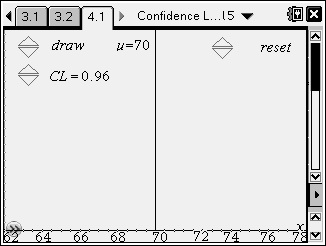# Activities

••• ##### Subject Area

• Math: Statistics: Confidence Intervals

• ##### Author9-12

45 Minutes

• ##### Device
• TI-Nspire™ Navigator™
• TI-Nspire™
• TI-Nspire™ CAS
• ##### Software

TI-Nspire™
TI-Nspire™ CAS

2.1

• ##### Report an Issue

Confidence Levels#### Activity Overview

Students will interpret a confidence level as the average success rate of the process used to produce an interval intended to contain the true mean of the population. They will recognize that as the confidence level increases, on average, the confidence interval increases in width.

#### Objectives

• Students will interpret a confidence level as the average success rate of the process used to produce an interval intended to contain the true mean of the population.
• Students will recognize that as the confidence level increases, on average, the confidence interval increases in width.

#### Vocabulary

• confidence interval
• confidence level
• population parameter

#### About the Lesson

• This lesson involves interpreting the meaning of a confidence level that is associated with a confidence interval. It assumes that students know what confidence intervals and have experience in calculating them.
• As a result, students will:
• Generate confidence intervals for the mean of a population using random samples of size n and investigate how often these intervals actually contain the true population mean.
• Use the actual population mean to check their estimates and to develop an understanding of the meaning of confidence level.
• Experiment with different confidence levels and finally investigate the relationship between confidence level and interval size.